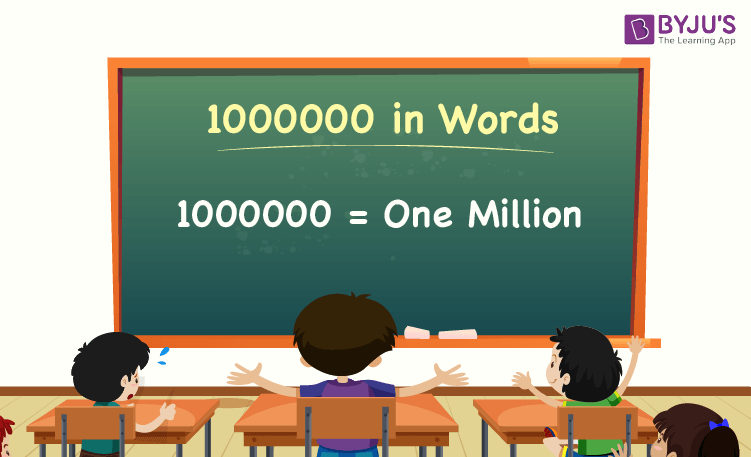# 1000000 in Words

1000000 in words is One Million. The number name of any number can be written using the ones, tens, hundreds, and thousands place of a number. Thus, the place value chart is helpful for writing the number 1000000 in words. For example, if you earned Rs. 1000000 in 10 years, you can write, “I have earned Rs. One Million in ten years”.

 1000000 in Words: One Million

In this article, let us discuss how to write 1000000 in words, and look at the solved examples in detail.

## How to Write 1000000 in Words?To write the number name of 1000000, identify the place value of each digit of the given number 1000000. Hence, for the number 1000000, the digit in one’s place is 0, the digit in ten’s place is 0, the digit in hundred’s place is 0, the digit in thousand’s place is 0, and the digit in million’s place is 1.

i.e.,1’s place of 1000000 is 0.

10’s place of 1000000 is 0.

100’s place of 1000000 is 0.

1000’s place of 1000000 is 0.

Million’s place of 1000000 is 1

Thus, the place value chart for 1000000 is:

 Millions Thousands Hundreds Tens Ones 1 0 0 0 0

Therefore, the number 1000000 in words is One Million.

### Examples

Example 1:

Find the value of 1200000 – 200000. Express the value in words.

Solution:

Given expression: 1200000 – 200000

⇒ 1200000 – 200000 = 1000000

So, the value of 1200000 – 200000 is 1000000.

Hence, 1000000 in words is One Million.

Example 2:

Express the value of One Million plus fifty thousand in words.

Solution:

One Million = 1000000

Fifty thousand = 50000

One Million plus fifty thousand = 1000000 + 50000 = 1050000

Hence, the value of one million plus fifty thousand is one million fifty thousand in words.

## Frequently Asked Questions on 1000000 in Words

### Write 1000000 in words.

1000000 in words is One Million.

### Express the value of 1000500 – 500 in words.

Simplifying 1000500 – 500, we get 1000000. Hence, 1000000 in words is One Million.

### How to write One Million in numbers?

One Million in numbers is 1000000.

### Is the number 1000000 an even number?

Yes, the number 1000000 is an even number.

### Is 1000000 a composite number?

Yes, 1000000 is a composite number.